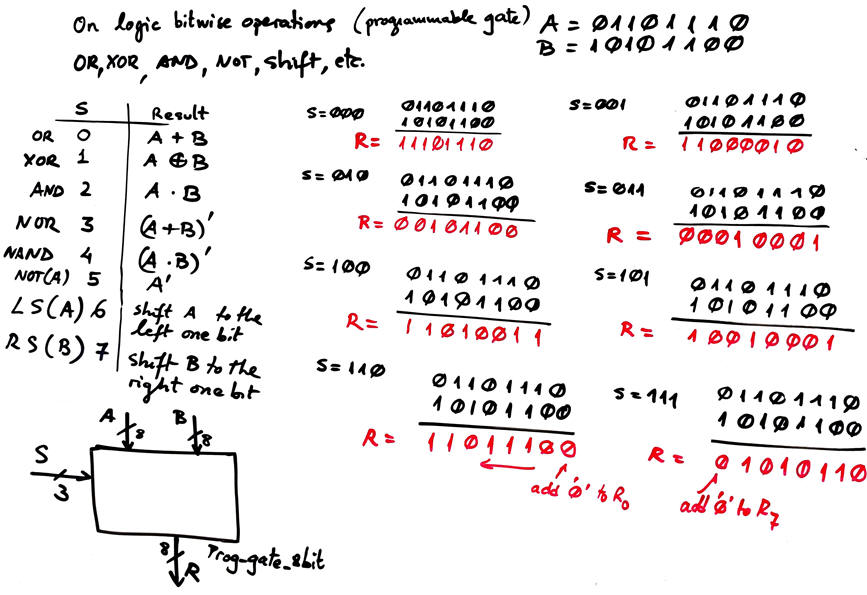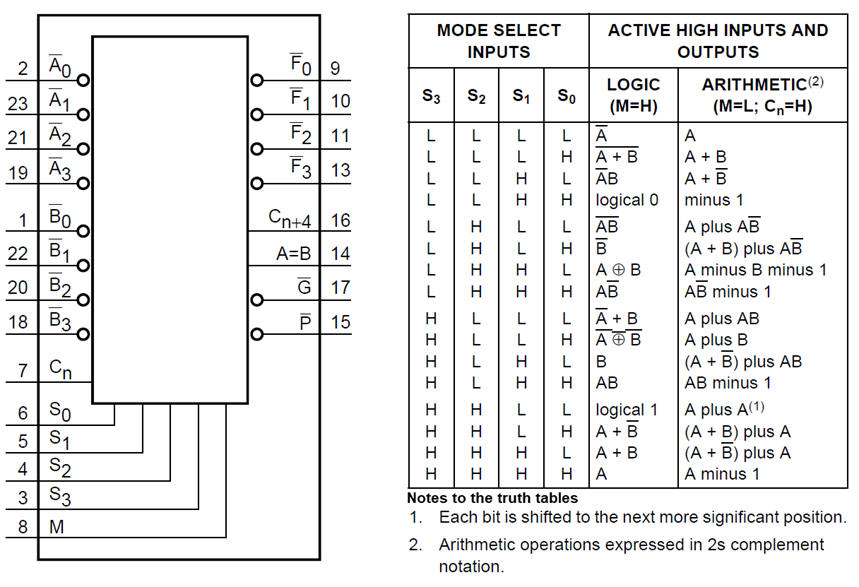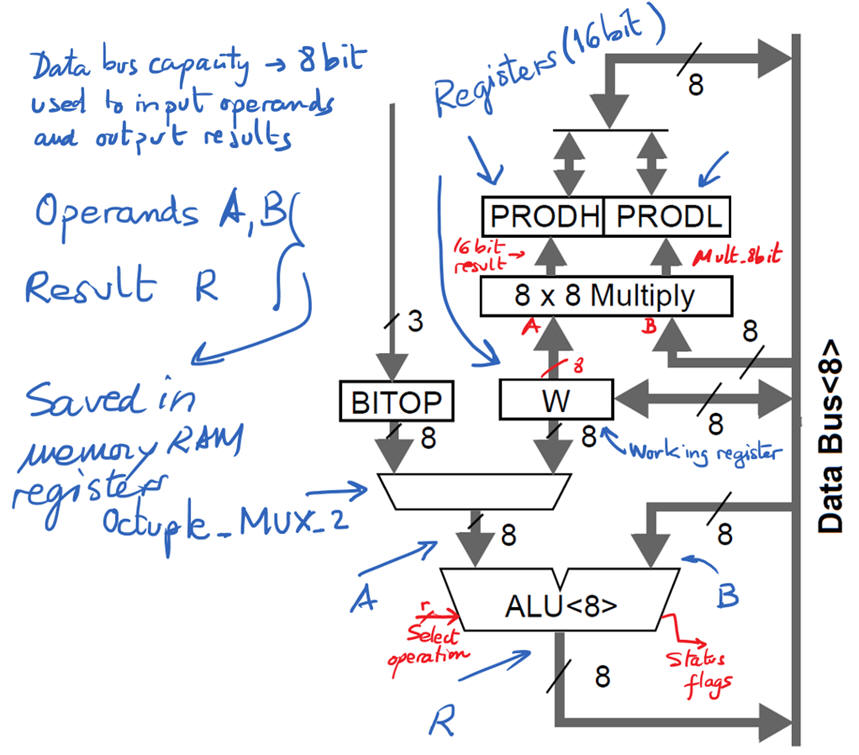﻿ Digital Circuits and Systems - Circuits i Sistemes Digitals (CSD) - EETAC - UPCBachelor's Degree in Telecommunications Systems and in Network EngineeringLecture 2 L4.2: Arithmetic and Logic Unit (ALU) [P2-P3-P4] Multifunctional circuits for logic and arithmetic processing [21/3]

1.11. Arithmetic Logic Unit (ALU_4bit)

1.11.1. Conceptual architecture

Arithmetic and logic unit architecture is modelled in this way, n-bit m operations can be selected using a multiplexer.1.11.2. Bitwise logic operations (AND, OR, etc.)This bitwise logic operations also introduces the concept of programmable or configurable gate.

Using an ALU and in a sequence of serialised operations, several computations can be performed with input operands.

For instance, if you have to perform an 8-bit subtraction in two's complement R = A - B , you can organise this sequence of operations, saving partial results R in memory positions  (data registers explained in next Chapter 2).

Algorithm to be solved: R = A - B = A + (-B) = A + 2C(B)

1) A = B  ; B = x (don't care) ; R = NOT(A)  (logic) --> and save partial result in Buf1

2) A  = Buf1  ;   B = 1 ;  R = A + B  (arithmetic)  --> and save result again in Buf1, so that it contains now the 2C(B)

3) A = A  ; B = Buf1 ;   R = A + B   (arithmetic)  ---> Thus R = A - B

1.11.3. Commercial standard arithmetic chips in classic technologies

The general architecture of an arithmetic and logic unit (ALU) performing several operations in parallel and then selecting only one result. However, real chips are optimised, in the same way we studied the Int_Add_Subt_8bit in previous lecture. Example classic chip: 74LS181. And this is a carry look-ahead generator 74HCT182 to which it can be combined to speed up operations.This is the internal block diagram on a microcontroller chip PIC18F4520, it contains an 8-bit ALU and also a hardware 8-bit multiplier.Status flags bits from ALU operations results (carry, decimal carry, sign, zero and overflow) are saved in a status register and can be used for making decisions in program flows.There are hundreds of web pages and videos over the internet on binary arithmetic circuits. And every on the subject has several chapters on arithmetic circuits because they are computer fundamental blocks.Dedicated processor representing the Arithmetic and Logic Unit (ALU) as the core of the datapath structure. Source: Hwang, E., Digital Logic and Microprocessor Design with VHDL, CL-Engineering, 2005.